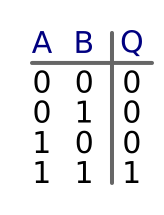# Truth tables

Martin McBride, 2017-01-15
Tags and gate 3-input gate truth table
Categories logic logic gates

A truth table is a way of representing the output of a logic gate for every possible set of inputs.

## 2 input gates

We have already seen the example of a 2 input AND gate, which has a truth table like this:Since there are 2 inputs (A and B), and each input has 2 possible states (0 or 1), there are 4 possible combinations of inputs: (0, 0), (0, 1), (1, 0) and (1, 1). In the case of an AND gate, the output is 0 unless both inputs are 1.

Notice that we arrange the combinations in a particular order. If you look down the columns:

• The values of B are 0, 1, 0, 1
• The values of A are 0, 0, 1, 1

## 3 input gates

It is possible for a gate to have more than 2 inputs. In the case of a gate with 3 inputs (A, B and C), there are 8 possible combinations (222). Here is the truth table for a 3 input and gateIn this case, the output is 0 unless all 3 inputs are 1.

Again, we arrange the combinations in a similar order. If you look down the columns:

• The values of C are 0, 1, 0, 1, 0, 1, 0, 1
• The values of B are 0, 0, 1, 1, 0, 0, 1, 1
• The values of A are 0, 0, 0, 0, 1, 1, 1, 1

Why do we use that particular order? You can think of the inputs as being numbers in binary:

• 000 binary is 0 denary
• 001 binary is 1 denary
• 010 binary is 2 denary
• 011 binary is 3 denary
• etc

So we are just placing the rows in numerical order.

## More inputs

It is possible to have logic circuits with 4 or more inputs, and we can simply extend the truth table to account for this.

For example, if there are 4 inputs (A, B, C and D), there will be 16 lines in the table. Extend the sequence of inputs using the same pattern.